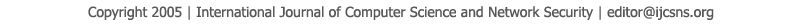To search, Click below search items.All Published Papers Search Service
 Title Author Year Keyword
 Title Comparing Three Different Estimators of fuzzy hazard Rate Function for Mixed Failure to Time Model Author Mahmod Mohamed Shakoor Amrir Citation Vol. 19  No. 12  pp. 43-46 Abstract This paper deals with constructing a new mixed probability failure model distribution, from mixing f1(x,?) and f2(x,?) were f1(x,?)is exponential and f2(x,?) is Gamma with (2,?), the mixing proportion are (p=α/(α+1)) and (1-p)=1/(α+1) , and after the p.d.f is constructing, we derive cumulative distribution function and reliability function, and also derive moments formula about origin. And then the two parameters (?,?) are estimated by maximum likelihood, and moments and proposed method, then we use (α ?,β ?) with fuzzy parameter (k ?_i) to compare different estimators of fuzzy hazard rate function, using different sets of initial values, and also of sample size (n), and the comparison is done through simulation. All results are explained in tables. Keywords fuzzy hazard rate Mixed Failure maximum likelihood estimated. URL http://paper.ijcsns.org/07_book/201912/20191207.pdf